Click to Chat

1800-1023-196

+91-120-4616500

CART 0

• 0

MY CART (5)

Use Coupon: CART20 and get 20% off on all online Study Material

ITEM
DETAILS
MRP
DISCOUNT
FINAL PRICE
Total Price: Rs.

There are no items in this cart.
Continue Shopping• Complete Physics Course - Class 11
• OFFERED PRICE: Rs. 2,968
• View Details

Fluid Statics

Table of Content

Fluid

Fluid Pressure

Variation of Pressure in a Stationary Fluid

Atmospheric Pressure

Variation of Pressure with Depth

Variation of Pressure along Horizontal in Accelerating Fluids

Pascal's Law

Simulation for Hydraulic Lift Works Principle

Related Resources

Fluid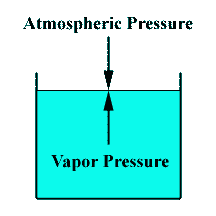A fluid is a substance, such as liquid or gas that has no rigidity like solids. Liquids are distinguished from gases by the presence of a surface. As fluids have no rigidity, they fail to support a shear stress. When a fluid is subjected to a shear stress, the layers of the fluid slide relative to each other. This characteristic gives the fluid, an ability to flow or change shapes.An object with less density than the fluid will float upward until it reaches the surface of the fluid. At that position, only part of the object is submerged.

Fluids obey Newton's laws of motion. Any infinitesimal volume of fluid accelerates according to net force acting on it.

Under static condition, the only force component that needs to be considered is one that acts normal or perpendicular to the surface of the fluid. No matter what the shape of a fluid, forces between the interior & exterior are at right angles to the fluid boundary.

The magnitude of normal force per unit surface area is called pressure. Pressure is a scalar quantity and has no directional properties.

The force  exerted by a fluid against the fluid surface depends on the pressure r according to

Here,  is the area vector (taken along the outward normal).

Fluid Pressure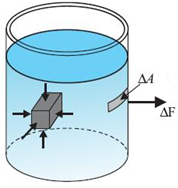Consider an elemental area dA inside a fluid, the fluid on one side of area dA presses the fluid on the other side and vice-versa. We define the pressure p a that point as the normal force per unit area, i.e.

p = dF⊥/dA

If the pressure is same at all the points of a finite plane surface with area A, then

p = F⊥/dA ; where F⊥ is the normal force on one side of the surface.

The SI unit of pressure is 'pascal'; where 1 pascal = 1 Pa = 1 N/m2

The pressure inside a fluid can be calculated by considering the following.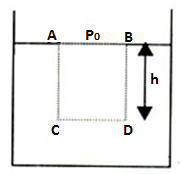If we analyse the force acting on surface CD we have to take into account the weight of the fluid column just above CD (other parts of the liquid cannot exert any force because liquid cannot exert shear stress).

Considering the pressure at the free surface to be p0, the force acting on the layer of fluid at CD = ρ0A + ρhAg, where ρ = density of liquid.

So, F= (ρ0 + ρgh)A

Hence, pressure acting at any point on CD =F/A=  (ρ0 + ρgh)/A

= ρ0 + ρgh.

Variation of Pressure in a Stationary Fluid

For any liquid of density ρ, the total pressure at a depth 'h' is given by P = Pressure at a liquid surface (p0) + Pressure due to liquid column.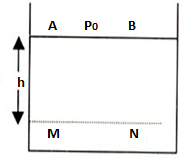P = p0 + ρgh

Total pressure at M, PM = p0 + ρgh

If the pressure at the surface (p0) is changed, an equal change in pressure is felt at all depths i.e. throughout the liquid.This phenomenon will be described while discussing Pascal's law.

Suppose the pressure at the liquid surface is altered by Δp, then the pressure at M is PM = p0' + hρg =

Where p0' = p0 + Δp

Then, PM = p0 + Δp + hρg

Refer this video to know more about fluid statics:-

Question:-

Does a fluid exert force on the surface of a container in which it is kept?

Fluids exert force on all surfaces in contact with them. They exert thrust in a direction normal to the surface in contact. The understand this, let us consider a surface in contact with a fluid. Let a be the area of the surface in contact. If the fluid pressure P on this area is same everywhere, then thrust on the surface F=PA along the normal to the surface.

So,Thrust due to fluid = ρghA = Vρg,

where V = Volume of the fluid.

Hence, the thrust on a surface depends on the volume of fluid above it. This suggests that if a body is immersed in a fluid the thrust on the lower surface will be more than that on the upper surface.

Atmospheric Pressure

It I the pressure of the earth's atmosphere. Normal atmospheric pressure at sea level (an average value) is 1 atmosphere (atm) which is equal to
1.013 × 105 Pa.

Fluid force acts perpendicular to any surface in the fluid, not matter how that surface is oriented. Hence, pressure has no intrinsic direction of its own, i.e. It is a scalar.

The excess pressure above atmospheric pressure is called gauge pressure, and total pressure is called absolute pressure

Variation of Pressure with Depth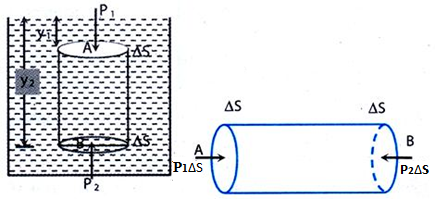(i) Let pressure at A is P1 and pressure at B is P2

Then, p2 ΔS = p1 ΔS + ρg ΔS (y2 - y1)

or p2 = p1 + ρg (y2 - y1)

The pressure increases with depth (y),

i.e. dp/dy = ρg; where ρ = density of the fluid (kg/m3).

(ii) Pressure is same at two points in the same horizontal level.

As body is in equilibrium, P1 ΔS = P2 ΔS

or P1 = P2

Variation of Pressure along Horizontal in Accelerating Fluids

(i) If the container is open at the top the shape of the liquid changes,

From equilibrium considerations:

The shaded element accelerates to the right.

=>p1 ΔS - p2 ΔS = mao = (ρl ΔS) ao                 ... (1)

p1 = h1 ρg + po; P2 = h2 ρg + Poor P1 - P2 = (h1 - h2) ρg

tan θ = h2 - h1/l                                         ... (2)

From (1),

(P1 - P2) = ρl ao

or (h1 - h2) ρg = ρl ao

or (h1 - h2) /l = ao/g

From (2),

tan θ = (ao/g)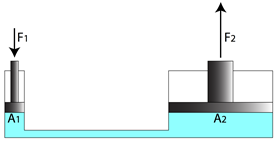(ii) If the container is completely filled and closed the pressure varies vertically as well as horizontally i.e.

dP/dy = -ρg and

dP/dx = -ρao

Pascal's Law

“Pascal's law states that when there is an increase in pressure at any point in a confined fluid, there is an equal increase at every other point in the container”.

Simulation for Hydraulic Lift Works Principle

This animations shows how a hydraulic lift works -in principle. This is an example of Pascal's Principle. Pressure equals force/area. The small force and small area on the pump side equals the large force and large area on the other side. The pressure is transferred equally through the hydraulics.

Question :-

Water is poured to same level in each of the vessels shown in the figure, all having the same base area. In which vessel is the force experienced by the base maximum?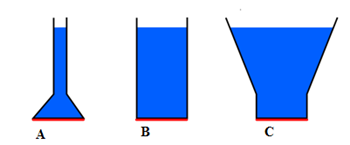Problem 1 :-A vertical U-tube of uniform cross-section contains mercury in both arms. A glycerine (relative density = 1.30 column of length 10 cm is introduced into one of the arms. Oil of density 800 kg m-3 is poured into the other arm until the upper surface of the oil and glycerine are the same horizontal level. Find the length of the oil column. Density of mercury is 13.6 × 103 kgm-3.

Solution:-

Pressure at A and B must be same.

Pressure at A = p0 + 0.1 × (1.3 × 1000) × g

Where p0 = atmospheric pressure

Pressure at B = p0 + h × 800 × g + (0.1 - h) × 13.6 × 1000 g

So, p0 + 0.1 × 1300 × g = po + 800 gh + 1360 g - 13600 × g × h

or h = 9.6 cm

Problem 2 :-For the arrangement shown in the figure, what is the density of oil?

Solution:-

psurface = po + ρw . gl

psurface = po + ρoil (l + d)g

=> ρoil = (ρω.l)/(l+d)

= 1000(135)/(135+12.3) = 916 kg/m3

A body of density r is released gently on the surface of a layer of liquid of depth 'd' and density s (r > s). Find out, after what time, it will reach the bottom of the liquid.

Solution:-

If V is volume of body, the weight of body

= mg = (Vr)g

Upthrust exerted by liquid on body = Vsg

Thus, Effective weight of body in liquid

Weff = Vrg - Vsg = V(r - s)g

Acceleration of body in liquid, a = Weff/m

= v(ρ-σ)g/vρ = (ρ-σ)/ρg

From relation h = ut + 1/2 at2

We get d = 0 + 1/2 at2

=> t = √2d/a

= [2d/{(ρ-σ)g/ρ}]½

= [2dρ/(ρ-σ)g]1/2

From the above observation we conclude that,  it will reach the bottom of the liquid after a time [2dρ/g(ρ-σ)]½.An ideal fluid is incompressible and nonviscous in nature.

Flow of ideal fluid is irrotational.This signifies, due to flow of fluid, no internal torques, causing rotational motion, can come into play.

Velocity of flow and pressure, at any point, do not change with time.

The velocity of any molecule, at a point, is independent of time.

Velocity of flow of liquid varies inveresely as the area of  cross section of the opening from where the liquid comes outQuestion 1 :-

In case of streamlined flow of liquid, the loss eneergy is:

(a) minimum          (b) maximum

(c) zero                 (d) infinite

Question 2 :-

What happens to viscosity of liquid and gas with the rise of temperature:

(a) viscosity of both decrease

(b) viscosity of both increase

(c) viscosity of liquid decreases but of gas increases

(d) viscosity of liquid increases but of gas decreases

Question 3 :-

The rain drops falling from the sky neither hit us nor make holes on the ground because they move with:

(a) constant acceleration            (b) variable acceleration

(c) variable speed                       (d) constant terminal speed

Question 4 :-

The velocity of falling rain drops attains limited value because of:

(a) upthrust of air               (b) viscous force exerted by air

(c) surface tension effects  (d) air currents in atmosphere

Question 5 :-

The clouds float in the atmosphere because of:

(a) their low temperature         (b) their low viscosity

(c) their low density                 (d) creation of low pressureQ.1
Q.2
Q.3
Q.4
Q.5

a

a

d

b

c

Related Resources

You might like to refer Solved Examples on Fluid Mechanics.

For getting an idea of the type of questions asked, refer the  Previous Year Question Papers.### Course Features

• 101 Video Lectures
• Revision Notes
• Previous Year Papers
• Mind Map
• Study Planner
• NCERT Solutions
• Discussion Forum
• Test paper with Video Solution